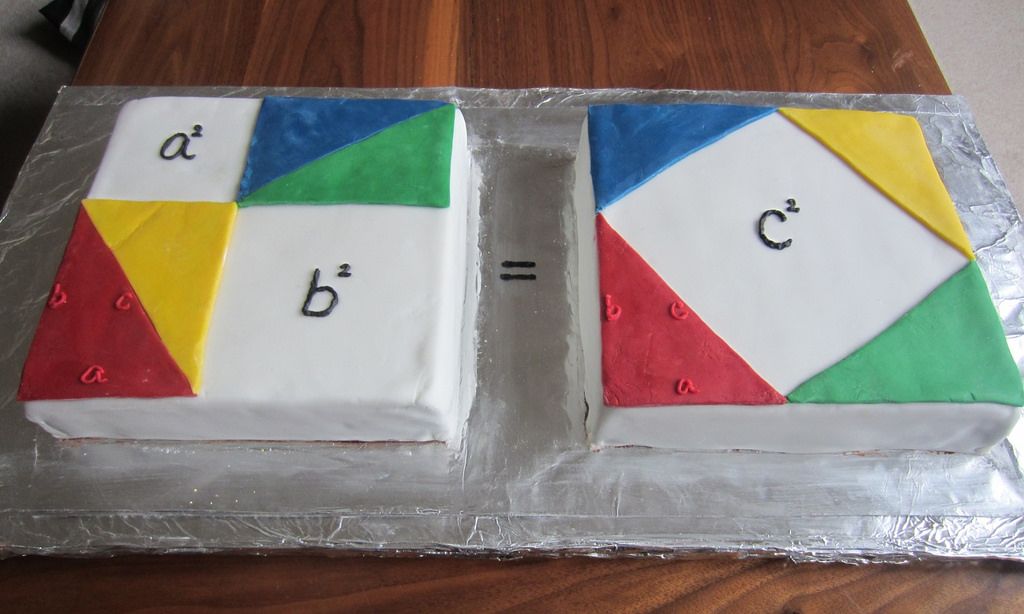### You have big data? MIT researchers can help shrinking it!

In a lot of machine learning and data science applications, it is not unusual to use matrices to represent data. It is indeed a very convenient way to keep the information but also to do manipulations, calculations and other useful tricks. As the size of the data increases, of course the size of the matrices grows too and that can be a bit problematic. Finding a way to reduce the size of these matrices while keeping the information is a challenge that a lot of us have faced. Using techniques that exploit the sparsity of the matrices, or even reducing the dimensionality via principal components is common practice.

Reading the latest World Economic Forum Newsletter I came to find out about a new algorithm that MIT researchers will present in the ACM Symposium on Theory of Computing in June. The algorithm is said to find the smallest possible approximation of an original matrix, guaranteeing reliable computations. Indeed the best way to determine how well de “reduced” matrix approximated the original one you need to measure the “distance” between them and a common distance to use the the typical Euclidean measure that we are all familiar with… What? You say you aren’t?… Remember Pythagoras?

The square of the hypotenuse (the side opposite the right angle) is equal to the sum of the squares of the other two sides.There you go… all you have to do is extend it to n-dimensions et voilà… That is not the only way to measure distance. Think for example the way in which you more in a grid-city such in Manhattan, New York City… You cannot take move diagonally (except in Broadway I suppose…) so you need to go north-sound or east-west. That distance is actually called the “Manhattan distance“.

Mathematicians refer to “norms” when talking about distance measurement and indeed both the Euclidean and Manhattan distances mentioned above are norms:

• Manhattan distance is a 1-norm measure, the sum of differences are raise to the power of 1.
• Euclidean distance is a 2-norm measure, the sum of differences are raise to the power of 2.
• etc…

So what about the MIT algorithm proposed by Richard Peng and Michael Cohen? Well they show that their algorithm is optimal for “reducing” matrices under any norm! The first step is to assign each row of the original matrix a “weight”. A row’s weight is related to the number of other rows that it is similar to. It also determines the likelihood that the row will be included in the reduced matrix.

Let us imagine that the row is indeed included in the reduced matrix. Then its values will be multiplied according to its weight. So, for instance, if 10 rows are similar to one another, but not to any other rows of the matrix, each will have a 10 percent chance of getting into the condensed matrix. If one of them does, its entries will all be multiplied by 10, so that it will reflect the contribution of the other nine rows.

You would think that using the Manhattan distance would be simpler than the Euclidian one when calculating the weights… Well you would be wrong! The previous best effort to reduce a matrix under the 1-norm would return a matrix whose number of rows was proportional to the number of columns of the original matrix raised to the power of 2.5. In the case of the Euclidean distance it would return a matrix whose number of rows is proportional to the number of columns of the original matrix times its own logarithm.

The MIT algorithm of Peng and Cohen is able to reduce matrices under the 1-norm as well as it does under the 2-norm. One important thing is that for the Euclidean norm, the reduction is as good as that of other algorithms… and that is because they use the same best algorithm out there… However, for the 1-norm it uses it recursively!

Interested in reading the paper? Well go to the ArXiV and take a look!